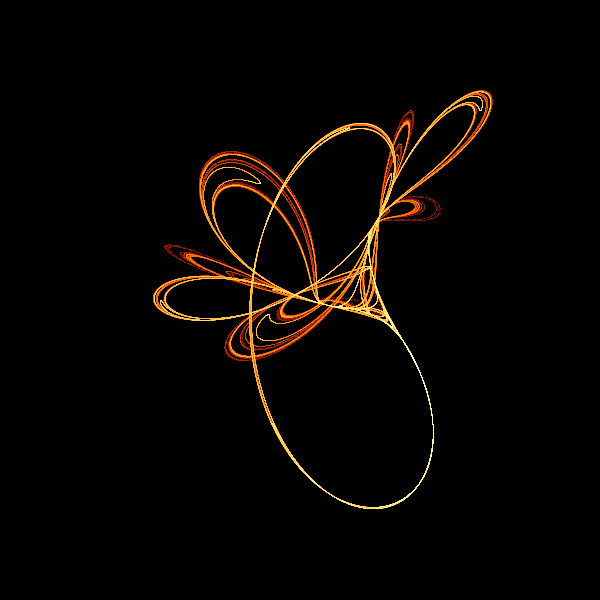# Tinkerbell fractal with generativepy

Martin McBride, 2020-11-01
Tags tinkerbell fractal
Categories generativepy generative art

generativepy has moved to the pythoninformer.com website, and this page might not be up to date. Please visit the new location.

The Tinkerbell fractal is based on an iterated function. Here we will look at an implementation using generativepy.

The basic algorithm is similar to the King's Dream fractal. It is best to read that article first, as this section mainly highlights the differences between them.

## Tinkerbell formula

The fractal equations for Tinkerbell are:

xnext = x*x - y*y + A*x + B*y
ynext = 2*x*y + C*x + D*y


Suitable parameters are:

A = 0.9
B = -0.6013
C = 2.0
D = 0.5

x = 0.01  # Initial value
y = 0.01  # Initial value


You can vary these values to create different variants of the fractal, although not all values will create pleasing images.

The code also modifies the scaling factors (in the Scaler constructor) to fit the size of the fractal.

Here is the result:## The code

Here is the full code for the image above:

from generativepy.bitmap import make_bitmap, Scaler
from generativepy.color import Color
import numpy as np
import math

MAX_COUNT = 10000000
BLACK = Color(0)
WHITE = Color(1)
A = 0.9
B = -0.6013
C = 2.0
D = 0.5

def build_color_table(size):
table = [Color.of_hsl(0.2*i/size, 1, 0.2+0.8*i/size) for i in range(size)]
table = BLACK
return table

def paint(image, pixel_width, pixel_height, frame_no, frame_count):
scaler = Scaler(pixel_width, pixel_height, width=3, startx=-2, starty=-2)
print(scaler.width)

counts = np.zeros([pixel_height, pixel_width], np.int32)

x = 0.01
y = 0.01
for i in range(MAX_COUNT):
x, y = x*x - y*y + A*x + B*y, 2*x*y + C*x + D*y
px, py = scaler.user_to_device(x, y)
counts[py, px] += 1

counts = np.log(counts + 1).astype(np.int)
max_count = np.max(counts)
colors = build_color_table(max_count+1)

for px in range(pixel_width):
for py in range(pixel_height):
col = colors[counts[py, px]]
image.putpixel((px, py), col.as_rgb_bytes())

make_bitmap('tinkerbell.png', paint, 600, 600)


## See also

See the iterated functions article for a list of other fractal examples.

Copyright (c) Axlesoft Ltd 2021